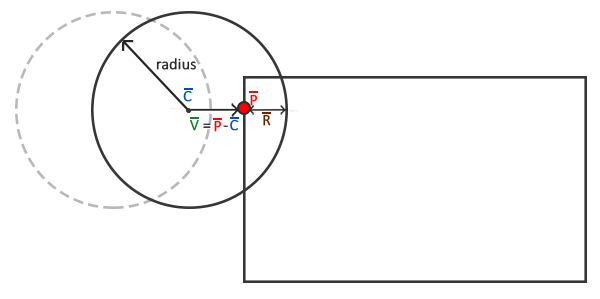# 碰撞处理

Note

### 碰撞重定位### 碰撞方向

1. 如果球撞击AABB的右侧或左侧，它的水平速度（x）将会反转。
2. 如果球撞击AABB的上侧或下侧，它的垂直速度（y）将会反转。

Direction VectorDirection(glm::vec2 target)
{
glm::vec2 compass[] = {
glm::vec2(0.0f, 1.0f),  // 上
glm::vec2(1.0f, 0.0f),  // 右
glm::vec2(0.0f, -1.0f), // 下
glm::vec2(-1.0f, 0.0f)  // 左
};
GLfloat max = 0.0f;
GLuint best_match = -1;
for (GLuint i = 0; i < 4; i++)
{
GLfloat dot_product = glm::dot(glm::normalize(target), compass[i]);
if (dot_product > max)
{
max = dot_product;
best_match = i;
}
}
return (Direction)best_match;
}


enum Direction {
UP,
RIGHT,
DOWN,
LEFT
};


### AABB - 圆碰撞检测

typedef std::tuple<GLboolean, Direction, glm::vec2> Collision;


Collision CheckCollision(BallObject &one, GameObject &two) // AABB - AABB 碰撞
{
[...]
return std::make_tuple(GL_TRUE, VectorDirection(difference), difference);
else
return std::make_tuple(GL_FALSE, UP, glm::vec2(0, 0));
}


Game类的DoCollision函数现在不仅仅只检测是否出现了碰撞，而且在碰撞发生时会有适当的动作。此函数现在会计算碰撞侵入的程度（如本教程一开始计时的示意图中所示）并且基于碰撞方向使球的位置矢量与其相加或相减。

void Game::DoCollisions()
{
for (GameObject &box : this->Levels[this->Level].Bricks)
{
if (!box.Destroyed)
{
Collision collision = CheckCollision(*Ball, box);
if (std::get<0>(collision)) // 如果collision 是 true
{
// 如果砖块不是实心就销毁砖块
if (!box.IsSolid)
box.Destroyed = GL_TRUE;
// 碰撞处理
Direction dir = std::get<1>(collision);
glm::vec2 diff_vector = std::get<2>(collision);
if (dir == LEFT || dir == RIGHT) // 水平方向碰撞
{
Ball->Velocity.x = -Ball->Velocity.x; // 反转水平速度
// 重定位
GLfloat penetration = Ball->Radius - std::abs(diff_vector.x);
if (dir == LEFT)
Ball->Position.x += penetration; // 将球右移
else
Ball->Position.x -= penetration; // 将球左移
}
else // 垂直方向碰撞
{
Ball->Velocity.y = -Ball->Velocity.y; // 反转垂直速度
// 重定位
GLfloat penetration = Ball->Radius - std::abs(diff_vector.y);
if (dir == UP)
Ball->Position.y -= penetration; // 将球上移
else
Ball->Position.y += penetration; // 将球下移
}
}
}
}
}


## 玩家 - 球碰撞

void Game::DoCollisions()
{
[...]
Collision result = CheckCollision(*Ball, *Player);
if (!Ball->Stuck && std::get<0>(result))
{
// 检查碰到了挡板的哪个位置，并根据碰到哪个位置来改变速度
GLfloat centerBoard = Player->Position.x + Player->Size.x / 2;
GLfloat distance = (Ball->Position.x + Ball->Radius) - centerBoard;
GLfloat percentage = distance / (Player->Size.x / 2);
// 依据结果移动
GLfloat strength = 2.0f;
glm::vec2 oldVelocity = Ball->Velocity;
Ball->Velocity.x = INITIAL_BALL_VELOCITY.x * percentage * strength;
Ball->Velocity.y = -Ball->Velocity.y;
Ball->Velocity = glm::normalize(Ball->Velocity) * glm::length(oldVelocity);
}
}


### 粘板

 //Ball->Velocity.y = -Ball->Velocity.y;
Ball->Velocity.y = -1 * abs(Ball->Velocity.y);


### 底部边界

void Game::Update(GLfloat dt)
{
[...]
if (Ball->Position.y >= this->Height) // 球是否接触底部边界？
{
this->ResetLevel();
this->ResetPlayer();
}
}


ResetLevelResetPlayer函数直接重新加载关卡并重置对象的各变量值为原始的值。现在游戏看起来应该是这样的：

## 一些注意事项

（我们使用的碰撞方案）仍然会出现这几个问题：

• 如果球运动得足够快，它可能在一帧内完整地穿过一个物体，而不会检测到碰撞。
• 如果球在一帧内同时撞击了一个以上的物体，它将会检测到两次碰撞并两次反转速度；这样不改变它的原始速度。
• 撞击到砖块的角时会在错误的方向反转速度，这是因为它在一帧内穿过的距离会引发VectorDirection返回水平方向还是垂直方向的差别。